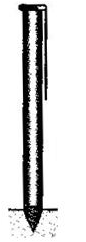Courses

# RD Sharma Test: Surface Area & Volumes

## 25 Questions MCQ Test Mathematics (Maths) Class 10 | RD Sharma Test: Surface Area & Volumes

Description
This mock test of RD Sharma Test: Surface Area & Volumes for Class 10 helps you for every Class 10 entrance exam. This contains 25 Multiple Choice Questions for Class 10 RD Sharma Test: Surface Area & Volumes (mcq) to study with solutions a complete question bank. The solved questions answers in this RD Sharma Test: Surface Area & Volumes quiz give you a good mix of easy questions and tough questions. Class 10 students definitely take this RD Sharma Test: Surface Area & Volumes exercise for a better result in the exam. You can find other RD Sharma Test: Surface Area & Volumes extra questions, long questions & short questions for Class 10 on EduRev as well by searching above.
QUESTION: 1

### The cost of painting a cubical box of side 3m at the rate of Rs.2 per sq.m is

Solution:

Given: Side of the cube (a) = 3 m
∴ Surface Area of Cube = 6a2 = 6 x 3 x 3 = 54 sq. cm
Now, Cost of painting the cubical box of 1 sq. m = Rs. 2 .
∴ Cost of painting the cubical box of 54 sq. m = 54 x 2 = Rs. 108

QUESTION: 2

### The volume of the cuboid whose length, breadth and height is 12cm, 8cm and 6cm is

Solution:

Volume of cuboid = Length x Breadth x Height
⇒ V = 12 x 8 x 6 = 576 cu. cm

QUESTION: 3

### The base area of the cylinder is 80 sq.cm. If its height is 5cm, then its volume is

Solution:

Base area of the cylinder = 80s.q cm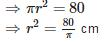Now, Volume of the cylinder =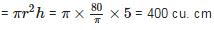QUESTION: 4

A cubical block of side 7 cm is surmounted by a hemisphere. The greatest diameter of the hemisphere is

Solution: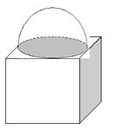It is clear that Maximum diameter of hemisphere can be the side of the cube.
∴ Greatest diameter of the hemisphere = 7 cm

QUESTION: 5

The plural form of ‘frustum’ is

Solution:

The plural form of ‘frustum’ is frusta.

QUESTION: 6

A solid cylinder of radius ‘r’ and height ‘h’ is placed over other cylinder of same height and radius. The total surface area of the shape so formed is

Solution: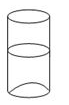Here,
TSA of new shape = 2πrh + 2πrh+ πr2
= 4πrh + 2πr

QUESTION: 7

The edge of the cube whose volume is 1728cm is

Solution:

Given : Volume of cube = 1728 cm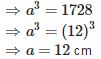QUESTION: 8

The radius and height of a right circular cone and that of a right circular cylinder are respectively equal. If the volume of the cylinder is 300 cu.cm, then the volume of the cone is

Solution:

Let Radius and height of the cone be r and h respectively and Radius and height of the right circular cylinder be R and H.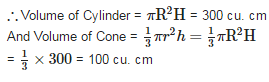QUESTION: 9

A solid is in the shape of a cone standing on a hemisphere with both their radii being equal to 1cm and the height of the cone is equal to its radius. The volume of the solid is

Solution: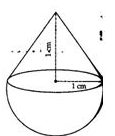Radii of cone and hemisphere = r = 3 cm
Height of cone (h) = 1 cm
Volume of solid = Volume of cone + Volume of hemisphere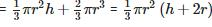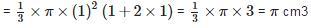QUESTION: 10

The length of the diagonal of a cuboid of dimensions 5cm by 4cm by 3cm is

Solution:

Length of the diagonal of cuboid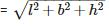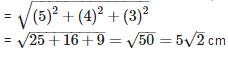QUESTION: 11

If two solid hemispheres of same base radius ‘x’ cm are joined together along their bases, then the CSA of the new solid formed is

Solution: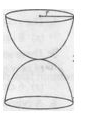If two solid hemispheres of same base radius ‘x’ cm are joined together along their bases, then the CSA of the new solid formed is 4πr2 = 4πx2.

QUESTION: 12

The volumes of two spheres are in the ratio 125 : 64. The ratio of their surface areas is

Solution:

Let r1 and r2 be the radius of the two spheres respectively. Therefore, the ratio of their surface areas,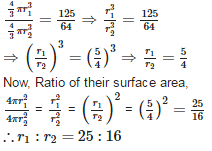QUESTION: 13

If the surface area of a sphere is 36π sq. cm, then its volume is

Solution:

Given: Surface area of sphere = 36πr sq. cm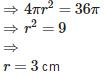∴ Volume of sphere =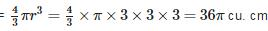QUESTION: 14

A frustum of a cone is of height 12cm with radii of its circular ends as 2cm and 4cm. The volume of the frustum is

Solution: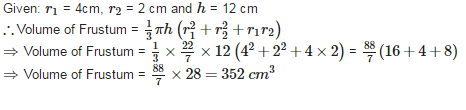QUESTION: 15

The longest rod that can be placed inside the cube is

Solution:

The longest rod that can be placed inside the cube is √3 times the edge of the cube, i.e., √3 edge

QUESTION: 16

If the surface area of the sphere is same as the CSA of a right circular cylinder whose height and diameter are 12cm each, then the radius of the sphere is

Solution:

Let the radius of solid sphere be rr cm and radius of solid cylinder be R cm. Then according to question,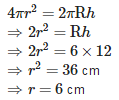QUESTION: 17

If the volume of a cube is 343 cm , then its edge is

Solution: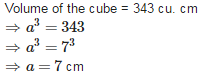QUESTION: 18

The inner dimensions of a closed box are 12cm, 10cm and 8cm. If the thickness of the wood is 1cm, then the capacity f the box is

Solution:

Given: l = 12 cm, b = 10 cm and h = 8 cm
∴ Capacity of a closed box = lbh = 12 x 10 x 8 = 960 cm. cm
Capacity of box of thickness 1 cm = 960/1 = 960 cu. cm

QUESTION: 19

A funnel is the combination of

Solution: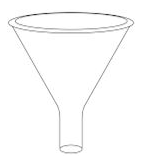A funnel is the combination of a frustum of a cone and a cylinder.

QUESTION: 20

A plumbline is combination of

Solution:

A plumbline is combination of a hemisphere and a cone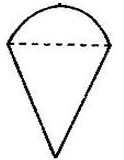QUESTION: 21

A rectangular piece of paper is 44cm long and 18cm wide. If a cylinder is formed by rolling the paper along its length, then the radius of the base of the cylinder is

Solution: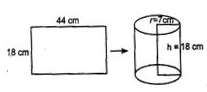Let r be the radius of the cylinder. Given: Circumference of cylinder = 44 cm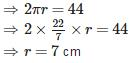QUESTION: 22

The surface areas of two spheres are in the ratio 16 : 9. The ratio of their volumes is

Solution:

Let r1 and r2 be the radius of the two spheres respectively. Therefore. the ratio of their volumes.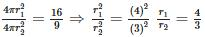Now, ratio of thier volumes,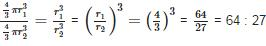QUESTION: 23

During conversion of a solid from one shape to another, the volume of the new shape will

Solution:

During conversion of a solid from one shape to another, the volume of the new shape will remain unaltered.

QUESTION: 24

The shape of a glass tumbler is usually in the form of

Solution: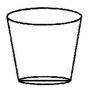The shape of a glass tumbler is usually in the form of frustum of a cone.

QUESTION: 25

A cylindrical pencil sharpened at one edge is the combination of

Solution:

A cylindrical pencil sharpened at one edge is the combination of a cone and a cylinder.